# Calculation rules - our fair algorithm for tracking results09.08.2018

How is the profit/loss calculated in the Safetrading.com reports?

Most of the Safetrading platform users have asked us how we calculate the percentage of profit or loss in the reports. We will answer this question and explain the calculations with help from the case of the Verified Crypto Telegram channel according to the results of Q2.

The report is as follows: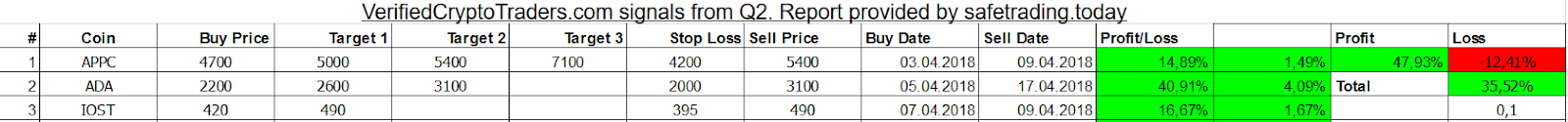The following designations are in the document: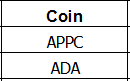Coin – the name of the coin/token to be purchased (from the signal)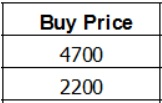Buy Price – the price, according to which the coin/token is purchased (from the signal)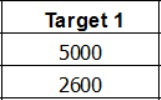Target - the price, according to which the sale of the coin during growth is recommended. If there are no targets in the signal, we leave the corresponding slots empty.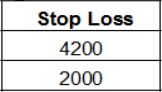Stop Loss – the price, according to which traders recommend selling the coin during fall to minimize loss (if the stop loss is not included in the signal, we leave the corresponding slot empty).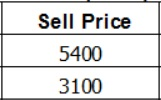Sell Price- the coin/token sale price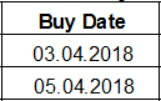Buy Date- the purchase date of the signal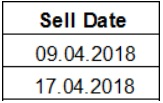Sell Date - the sale date of the coin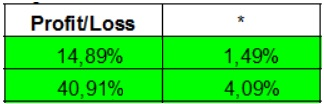Profit/loss - in this column, we state by how many percentage points the coin/token grew compared to the buy price.

*Note! We state by how many percentage points you would have increased your profit had you invested 10% of your deposit/portfolio into the signal. You can find the details below.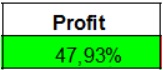Profit - it is a general sum of profit from all the signals over the reporting period.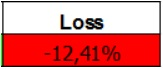Loss - it is a general sum of loss from all the signals over the reporting period.

With the help of the following example we will review the stats calculation in detail:

To make the calculations simple, let’s imagine that the whole deposit/portfolio is equal to 1 BTC. The most important thing is that when we do our calculations, we assume that each signal is purchased for the amount of 10% of the deposit. This is a general rule for all traders, as it ensures risk minimization. Sometimes these numbers can vary between 10-25%, but we took the average number, 10%, for all the providers.

Let’s review the case with stop losses and targets in the signals:

Let’s take a look at the first signal ADA.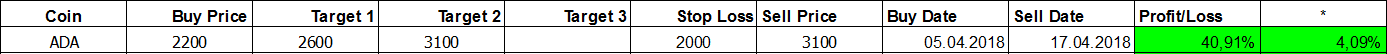It was purchased for a price of 2,200 Satoshi, reached the second target of 3100 Satoshi on 05.04.2018, and was sold on 17.04.2018. As a result, the general profit according to this coin is 40.91% compared to the purchase price, and 4.09% as compared to the total deposit amount (1 BTC).

Let’s take a look at the second MOD signal now.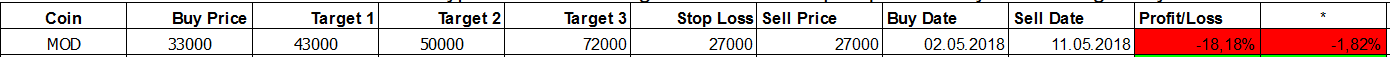It was purchased for a price of 33,000 Satoshi, reached stop loss on 02.05.2018, reached stop loss of 27,000 Satoshi, and was sold on 11.05.2018. As a result, general loss according to this coin is -18.18% compared to the purchase price, and -1.82% as compared to the total deposit amount. Total profit according to the two coins is 2.27%, with respect to the whole portfolio.

Let’s take a look at the last coin, WABI.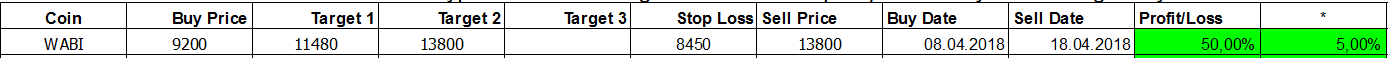It was purchased at the price of 9200 Satoshi on 08.04.2018, reached the second target of 13800 Satoshi and was sold on 18.04.2018. This way, general profit according to this coin is 50% compared to the purchase price and 5% compared to the total deposit amount. So, total profit according to the three coins is 7,27% with respect to the whole portfolio.

Let’s take a look at a case without any stop losses and targets in the signals.

A major difference between signals with targets and stop losses is that, in our calculations, we assume that the coin is sold at the moment of its maximum value over the reporting period. As well, if the stop loss was not stated in the signal, and no further instructions regarding the discussed purchase were given, we keep the token until growth starts, or sell it according to the current price during the last day of the reporting period (whichever comes first).

Let’s take a look at the first signal from the report of Infocrypto provider: LUX.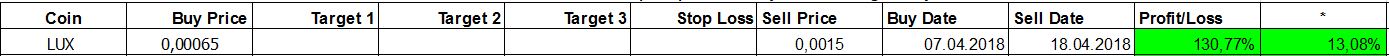It was purchased for a price of 0.00065 BTC on 07.04.2018, reached the maximum of 0.0015 BTC and was sold on 18.04.2018. As a result, the total profit for this coin was 130.77% of the coin purchase price, but only 13.08% of the total deposit amount.

Let’s take a look at the second signal AEON.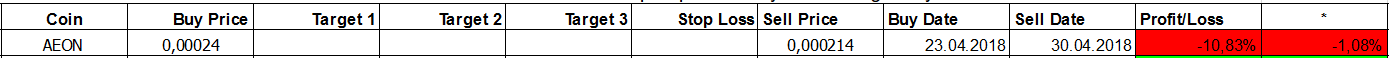It was purchased for a price of 0.00024 BTC on 23.04.2018, the coin did not grow until the end of the reporting period and was sold on the last day of the reporting period for a  price of 0.000214 BTC. As a result, the general loss according to this coin was -10.83% of the coin purchase price, and -1.08% of the total amount of the deposit. General profit according to the two coins was 12% of the total deposit.

According to our principle, the coin is sold automatically on the last day of the reporting period regardless of the behavior of the coin in future. In a specific case, we can review the results of the automatically sold coin upon the request of the provider.

What conclusions can we make?

As we have already mentioned, we use average data and calculate potential profit from the signals, assuming that you spend 10% of your deposit on every signal.

Outstanding Cases: We will add unique cases here.

When are the reports calculated? We calculate the reports every two months and publish them at the beginning of the following (third) month. For example, the reports for January and February were published at the beginning of March. The reports for March and April were published in May, and so on.

### 10 Best Crypto Providers 2020 | safetrading.today

30.11.2019

In today’s crypto signals review we are going to discuss 10 providers that have already proved their professionalism, attention to subscribers and a wide...